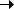# Number

 Module ejs Definition final class Number Inheritance NumberObject Stability Evolving.

The Number type is used by all numeric values in Ejscript.

Depending on how Ejscript is configured, the underlying number representation may be based on either an int, long, int64 or double data type. If the underlying type is integral (not double) then some of these routines may not be relevant.

## Properties

QualifiersPropertyTypeDescription
static const MAX_VALUENumberReturn the maximim value this number type can assume. Alias for MaxValue. An object of the appropriate number with its value set to the maximum value allowed.
static const MIN_VALUENumberReturn the minimum value this number type can assume. Alias for MinValue. An object of the appropriate number with its value set to the minimum value allowed.
static const MaxValueNumberReturn the maximim value this number type can assume.
static const MinValueNumberReturn the minimum value this number type can assume.
static const NEGATIVE_INFINITYNumberReturn a unique value which is less than or equal then any value which the number can assume.
static const NaNNumberNot a Number. This is the result of an arithmetic expression which has no value.
static const POSITIVE_INFINITYNumberReturn a unique value which is greater then any value which the number can assume.
get absNumberThe absolute value of a number (which is equal to its magnitude).
get byteNumberByte sized integral number. Numbers are rounded and truncated as necessary.
get ceilNumberThe smallest integral number that is greater or equal to the number value.
get floorNumberThe largest integral number that is smaller than the number value.
get isFiniteBooleanIs the number is not Infinity or -Infinity. This is set to true or false.
get isNaNBooleanIs the number equal to the NaN value. If the numeric type is integral, this will always return false.
get roundNumberIntegral number that is closest to this number. Ie. rounded up or down to the closest integer.

## Number Class Methods

(No own class methods defined)

QualifiersMethod

## Number Instance Methods

QualifiersMethod
Number(value: Object = null)
Number constructor.
iterator override get(): Iterator
Return an iterator that can be used to iterate a given number of times.
integral(size: Number = 32): Number
Convert this number to an integral value of the specified number of bits.
iterator override getValues(): Iterator
Return an iterator that can be used to iterate a given number of times.
max(other: Array): Number
Returns the greater of the number and the arguments.
toExponential(fractionDigits: Number = 0): String
Returns the number formatted as a string in scientific notation with one digit before the decimal point and the argument number of digits after it.
toFixed(fractionDigits: Number = 0): String
Returns the number formatted as a string with the specified number of digits after the decimal point.
min(other: Array): Number
Returns the lessor of the number and the arguments.
power(nth: Number): Number
Returns a number which is equal to this number raised to the power of the argument.
toPrecision(numDigits: Number = MAX_VALUE): String
Returns the number formatted as a string in either fixed or exponential notation with argument number of digits.
override toString(radix: Number = 10): String
This function converts the number to a string representation.

## Method Detail

Number(value: Object = null)
Description
Number constructor.
Parameters
 value: Object Value to use in creating the Number object. If the value cannot be converted to a number, the value will ba NaN (or 0 if using integer numerics). 8. [default: null]

override iterator get(): Iterator
Description
Return an iterator that can be used to iterate a given number of times. This is used in for/in statements.
Returns
An iterator.
Specified
ejscript-2.5
Example
```for (i in 5)
print(i)
@spec ejs```

integral(size: Number = 32): Number
Description
Convert this number to an integral value of the specified number of bits. Floating point numbers are converted to integral values using truncation.
Parameters
 size: Number null [default: 32]
Returns
An integral number.
Specified
ejscript-2.5

override iterator getValues(): Iterator
Description
Return an iterator that can be used to iterate a given number of times. This is used in for/each statements.
Returns
An iterator.
Specified
ejscript-2.5
Example
```for each (i in 5)
print(i)
@spec ejs```

max(other: Array): Number
Description
Returns the greater of the number and the arguments.
Parameters
 other: Array Other numbers to compare with.
Returns
A number.
Specified
ejscript-2.5

toExponential(fractionDigits: Number = 0): String
Description
Returns the number formatted as a string in scientific notation with one digit before the decimal point and the argument number of digits after it.
Parameters
 fractionDigits: Number The number of digits in the fraction. [default: 0]
Returns
A string representing the number.

toFixed(fractionDigits: Number = 0): String
Description
Returns the number formatted as a string with the specified number of digits after the decimal point.
Parameters
 fractionDigits: Number The number of digits in the fraction. [default: 0]
Returns
A string representing the number.

min(other: Array): Number
Description
Returns the lessor of the number and the arguments.
Parameters
 other: Array Numbers to compare with.
Returns
A number.
Specified
ejs 0

power(nth: Number): Number
Description
Returns a number which is equal to this number raised to the power of the argument.
Parameters
 nth: Number Nth Power to be raised to.
Returns
A number.
Specified
ejs 0

toPrecision(numDigits: Number = MAX_VALUE): String
Description
Returns the number formatted as a string in either fixed or exponential notation with argument number of digits.
Parameters
 numDigits: Number The number of digits in the result. If omitted, the entire number is returned. [default: MAX_VALUE]
Returns
A string.

override toString(radix: Number = 10): String
Description
This function converts the number to a string representation.
Parameters
 radix: Number Radix to use for the conversion. Defaults to 10. Non-default radixes are currently not supported. [default: 10]
Returns
A string representation of the number.